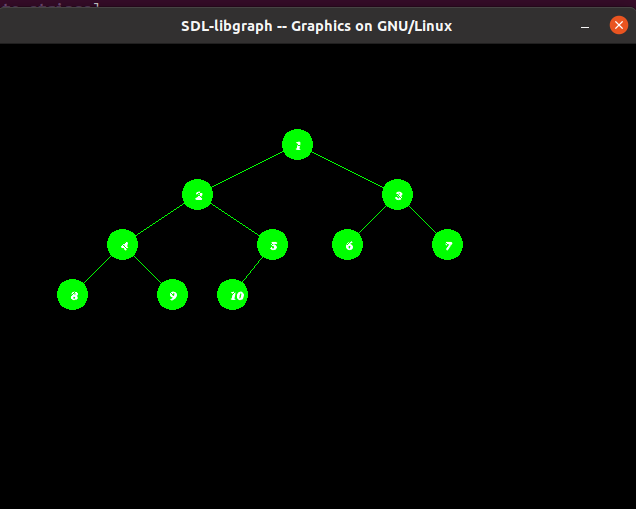# Represent Tree using graphics in C/C++

• Difficulty Level : Hard
• Last Updated : 05 May, 2021

Prerequisite: graphics.h, How to include graphics.h?

In C/C++ there is graphics.h header file which is used to create the object like line, circle, etc.
Given an array arr[] of N integers, the task is to write C++ program to create the Tree using graphics.h.

Want to learn from the best curated videos and practice problems, check out the C++ Foundation Course for Basic to Advanced C++ and C++ STL Course for the language and STL. To complete your preparation from learning a language to DS Algo and many more, please refer Complete Interview Preparation Course.

Approach: To run the program we have the include the below header file:

`#include `

We will create a Tree with the help below functions:

1. setcolor(color): This function present in graphic.h header file which is used to set the current drawing color to the new color.
2. floodfill(pattern, color): function is used to fill an enclosed area. The current fill pattern and fill color is used to fill the area.
3. circle(x, y, radius): The header file graphics.h contains circle() function which draws a circle with center at (x, y) and given radius.
4. outtextxy(): The header file graphics.h contains outtextxy() function which displays the text or string at a specified point (x, y) on the screen.

Below is the implementation of to draw Tree using graphics in C++:

## C++

 `// C++ program to draw the tree``// in graphics.h``#include ``#include ``#include ``#include ``using` `namespace` `std;` `// Function that prints Tree using``// functions graphic.h header file``void` `printTree(``int` `x, ``int` `y, ``int``* array,``               ``int` `index,``               ``int` `total_elements)``{` `    ``// Base Case``    ``if` `(index >= total_elements)``        ``return` `NULL;` `    ``// Convert int value into string``    ``ostringstream str1;``    ``str1 << array[index];` `    ``string str2 = str1.str();``    ``char``* str = &str2[0u];` `    ``// Set color of the boundary of``    ``// circle as green``    ``setcolor(GREEN);` `    ``// Draw the circle of radius 15``    ``// that represent node of Tree``    ``circle(x, y, 15);``    ``floodfill(x, y, GREEN);` `    ``// Print the values of the node``    ``// in the circle``    ``outtextxy(x - 2, y - 3, str);` `    ``// Set the color of the line``    ``// from parent to child as green``    ``setcolor(GREEN);` `    ``// Evaluating left and right child``    ``int` `left = 2 * index + 1;``    ``int` `right = 2 * index + 2;` `    ``// Recursively draw the left subtree``    ``// and the right subtree``    ``printTree(x - y / (index + 1), y + 50,``              ``array, left, total_elements);` `    ``printTree(x + y / (index + 1), y + 50,``              ``array, right, total_elements);` `    ``// Draw the line (or link) when the``    ``// node is not the leaf node``    ``if` `(left < total_elements) {``        ``line(x, y, x - y / (index + 1), y + 50);``    ``}` `    ``if` `(right < total_elements) {``        ``line(x, y, x + y / (index + 1), y + 50);``    ``}` `    ``return` `NULL;``}` `// Driver Code``int` `main()``{``    ``// Initialize graphic driver``    ``int` `gd = DETECT, gm;``    ``initgraph(&gd, &gm, ``"None"``);` `    ``// Consider the tree as represented``    ``/*``             ``1``          ``/     \``         ``2       3``       ``/  \     / \``      ``4   5    6   7``     ``/ \  /``     ``8  9 10``    ``*/` `    ``// Given array arr[]``    ``int` `array[] = { 1, 2, 3, 4, 5,``                    ``6, 7, 8, 9, 10 };` `    ``// Function Call``    ``printTree(300, 100, array, 0, 10);``    ``getch();` `    ``// closegraph function closes the``    ``// graphics mode and deallocates``    ``// all memory allocated by``    ``// graphics system``    ``closegraph();``}`

Output:My Personal Notes arrow_drop_up